# 8.3 Two-Dimensional Jacobi Example with One-Dimensional Decomposition

## 8.3 Two-Dimensional Jacobi Example with One-Dimensional Decomposition

A common use of parallel computers in scientific computation is to approximate the solution of a partial differential equation (PDE). One of the most common PDEs, at least in textbooks, is the Poisson equation (here shown in two dimensions):

 (8.1)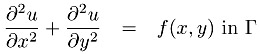(8.2)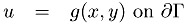This equation is used to describe many physical phenomena, including fluid flow and electrostatics. The equation has two parts: a differential equation applied everywhere within a domain F (8.1) and a specification of the value of the unknown u along the boundary of Γ (the notation ∂Γ means "the boundary of Γ"). For example, if this equation is used to model the equilibrium distribution of temperature inside a region, the boundary condition g(x, y) specifies the applied temperature along the boundary, f(x, y) is zero, and u(x, y) is the temperature within the region. To simplify the rest of this example, we will consider only a simple domain Γ consisting of a square (see Figure 8.8).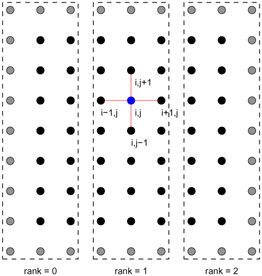Figure 8.8: Domain and 9 9 computational mesh for approximating the solution to the Poisson problem.

To compute an approximation to u(x, y), we must first reduce the problem to finite size. We cannot determine the value of u everywhere; instead, we will approximate u at a finite number of points (xi,yj) in the domain, where xi = i h and yj = j h. (Of course, we can define a value for u at other points in the domain by interpolating from these values that we determine, but the approximation is defined by the value of u at the points (xi,yj).) These points are shown as black disks in Figure 8.8. Because of this regular spacing, the points are said to make up a regular mesh. At each of these points, we approximate the partial derivatives with finite differences. For example,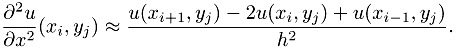If we now let ui,j stand for our approximation to solution of Equation 8.1 at the

point (xi, yj), we have the following set of simultaneous linear equations for the values of u:

 (8.3)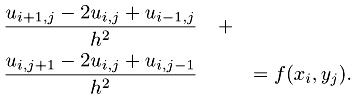For values of u along the boundary (e.g., at x = 0 or y = 1), the value of the boundary condition g is used. If h = l/(n + 1) (so there are n n points in the interior of the mesh), this gives us n2 simultaneous linear equations to solve.

Many methods can be used to solve these equations. In fact, if you have this particular problem, you should use one of the numerical libraries described in Section 12.2. In this section, we describe a very simple (and inefficient) algorithm because, from a parallel computing perspective, it illustrates how to program more effective and general methods. The method that we use is called the Jacobi method for solving systems of linear equations. The Jacobi method computes successive approximations to the solution of Equation 8.3 by rewriting the equation as follows:

 (8.4)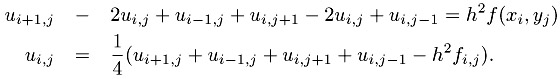Each step in the Jacobi iteration computes a new approximation to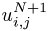in terms of the surrounding values of uN:

 (8.5)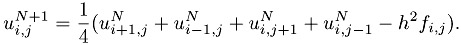This is our algorithm for computing the approximation to the solution of the Poisson problem. We emphasize that the Jacobi method is a poor numerical method but that the same communication patterns apply to many finite difference, volume, or element discretizations solved by iterative techniques.

In the uniprocessor version of this algorithm, the solution u is represented by a two-dimensional array u[max_n] [max_n], and the iteration is written as follows:

`    double u[NX+2][NY+2], u_new[NX+2][NY+2], f[NX+2][NY+2];    int    i, j;    ...    for (i=1;i<=NX;i++)       for (j=1;j<=NY;j++)          u_new[i][j] = 0.25 * (u[i+1][j] + u[i-1][j] +                                u[i][j+1] + u[i][j-l] - h*h*f[i][j]); `

Here, we let u[j], u[n+1][j], u[i], and u[i][n+1] hold the values of the boundary conditions g (these correspond to u(0,y), u(1, y), u(x, 0), and u(x, 1) in Equation 8.1). To parallelize this method, we must first decide how to decompose the data structure u and u_new across the processes. Many possible decompositions exist. One of the simplest is to divide the domain into strips as shown in Figure 8.8.

Let the local representation of the array u be ulocal; that is, each process declares an array ulocal that contains the part of u held by that process. No process has all of u; the data structure representing u is decomposed among all of the processes. The code that is used on each process to implement the Jacobi method is

`    double ulocal_new[NLOCAL][NY+2];    ...    for (i=i_start;i<=i_end;i++)      for (j=1;j<=NY;j++)        ulocal_new[i-i_start][j] =           0.25 * (ulocal[i-i_start+1][j] + ulocal[i-i_start-1][j] +                   ulocal[i-i_start][j+1] + ulocal[i-i_start][j-1] -                   h*h*flocal[i-i_start][j]); `

where i_start and i_end describe the strip on this process (in practice, the loop would be from zero to i_end-i_start; we use this formulation to maintain the correspondence with the uniprocessor code). We have defined ulocal so that ulocal[j] corresponds to u[i_start][j] in the uniprocessor version of this code. Using variable names such as ulocal that make it obvious which variables are part of a distributed data structure is often a good idea.

From this code, we can see what data we need to communicate. For i=i_start we need the values of u[i_start-1][j] for j between 1 and NY, and for i=i_end we need u[i_end+1][j] for the same range of j. These values belong to the adjacent processes and must be communicated. In addition, we need a location in which to store these values. We could use a separate array, but for regular meshes the most common approach is to use ghost or halo cells, where extra space is set aside in the ulocal array to hold the values from neighboring processes. In this case, we need only a single column of neighboring data, so we will let u_local[j] correspond to u[i_start][j]. This changes the code for a single iteration of the loop to

`    exchange_nbrs( ulocal, i_start, i_end, left, right );    for (i_local=1; i_local<=i_end-i_start+1; i_local++)      for (j=1; j<=NY; j++) `

`       ulocal_new[i_local][j] =          0.25 * (ulocal[i_local+1][j] + ulocal[i_local-1][j] +                  ulocal[i_local][j+1] + ulocal[i_local][j-1] -                  h*h*flocal[i_local][j]); `

where we have converted the i index to be relative to the start of ulocal rather than u. All that is left is to describe the routine exchange_nbrs that exchanges data between the neighboring processes. A very simple routine is shown in Figure 8.9.` void exchange_nbrs( double ulocal[][NY+2], int i_start, int i_end,                     int left, int right ) {     MPI_Status status;     int c;     /* Send and receive from the left neighbor */     MPI_Send( &ulocal, NY, MPI_DOUBLE, left, 0,               MPI_COMM_WORLD );     MPI_Recv( &ulocal, NY, MPI_DOUBLE, left, 0,               MPI_COMM_WORLD, &status );     /* Send and receive from the right neighbor */     c = i_end - i_start + 1;     MPI_Send( &ulocal[c], NY, MPI_DOUBLE, right, 0,               MPI_COMM_WORLD );     MPI_Recv( &ulocal[c+1], NY, MPI_DOUBLE, right, 0,               MPI_COMM_WORLD, &status ); } `Figure 8.9: A simple version of the neighbor exchange code. See the text for a discussion of the limitations of this routine.

We note that ISO/ANSI C (unlike Fortran) does not allow runtime dimensioning of multidimensional arrays. To keep these examples simple in C, we use compile-time dimensioning of the arrays. An alternative in C is to pass the arrays as one-dimensional arrays and compute the appropriate offsets.

The values left and right are used for the ranks of the left and right neighbors, respectively. These can be computed simply by using the following:

`     int rank, size, left, right;     ... `

`     MPI_Comm_rank( MPI_COMM_WORLD, &rank );     MPI_Comm_size( MPI_COMM_WORLD, &size  );     left = rank - 1;     right = rank + 1;     if (left < 0)      left  = MPI_PROC_NULL;     if (right >= size) right = MPI_PROC_NULL; `

The special rank MPI_PROC_NULL indicates the edges of the mesh. If MPI_PROC_NULL is used as the source or destination rank in an MPI communication call, the operation is ignored. MPI also provides routines to compute the neighbors in a regular mesh of arbitrary dimension and to help an application choose a decomposition that is efficient for the parallel computer.

The code in exchange_nbrs will work with most MPI implementations for small values of n but, as described in Section 9.3, is not good practice (and will fail for values of NY greater than an implementation-defined threshold). A better approach in MPI is to use the MPI_Sendrecv routine when exchanging data between two processes, as shown in Figure 8.10.` /* Better exchange code.  */ void exchange_nbrs( double ulocal[][NY+2], int i_start, int i_end,                     int left, int right ) {     MPI_Status status;     int c;     /* Send and receive from the left neighbor */     MPI_Sendrecv( &ulocal, NY, MPI_DOUBLE, left, 0,                   &ulocal, NY, MPI_DOUBLE, left, 0,                   MPI_COMM_WORLD, &status );     /* Send and receive from the right neighbor */     c = i_end - i_start + 1;     MPI_Sendrecv( &ulocal[c], NY, MPI_DOUBLE, right, 0,                   &ulocal[c+1], NY, MPI_DOUBLE, right, 0,                   MPI_COMM_WORLD, &status ); } `Figure 8.10: A better version of the neighbor exchange code.

In Sections 9.3 and 9.7, we discuss other implementations of the exchange routine that can provide higher performance. MPI support for more scalable decompositions of the data is described in Section 9.3.2.Beowulf Cluster Computing With Linux 2003
ISBN: N/A
EAN: N/A
Year: 2005
Pages: 198